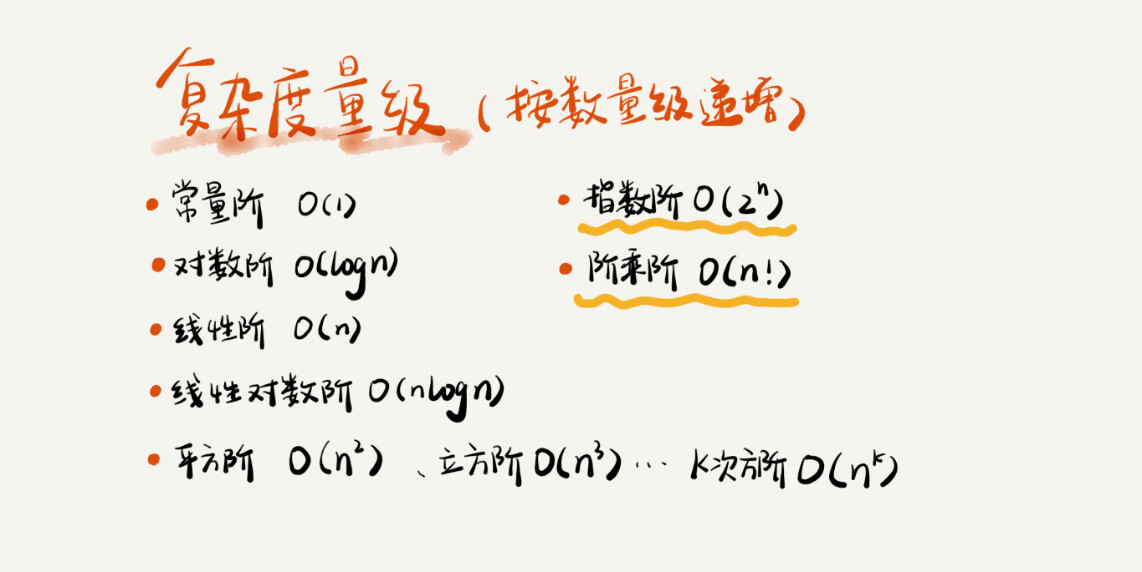XUHAO’S 博客 Always hungry to keep learning.

# 复杂度分析（上）：如何分析、统计算法的执行效率和资源消耗?

Tip：各平台的 markdown 解析标准不同，会有些数学符号无法识别，比如^n^: 表示n次方，~y~: 表示y 的底数。

# 为什么需要复杂度分析？

1. 测试结果非常依赖测试环境 测试环境中硬件的不同会对测试结果有很大的影响。比如，我们拿同样一份代码，分别在 Intel Core i9处理器和 Intel Core i3处理器来运行，肯定 i9比 i3处理器快的多，同样一份代码，在不同的机器上，执行的速度会有不同。
2. 测试接口受数据规模的影响很大 以排序算法为例，同一个排序算法，待排序数据的有序度不一样，排序的执行时间就会有很大的差别。极端情况，如果数据已经是有序的，那排序算法的不需要做任何操作，执行时间就会很短，如果测试数据规模调小，测试结果可能无法真实地反应算法的性能，比如，对于小规模的数据排序，插入排序可能反倒会比快速排序要快！

# 如何进行复杂度分析？

## 大 O 复杂度表示法

 int cal(int n) {
int sum = 0;
int i = 1;
for (; i <= n; ++i) {
sum = sum + i;
}
return sum;
}


 int cal(int n) {
int sum = 0;
int i = 1;
int j = 1;
for (; i <= n; ++i) {
j = 1;
for (; j <= n; ++j) {
sum = sum +  i * j;
}
}
}


   T(n) = O(n) ;  T(n) = O(n^2^)。


## 时间复杂度分析

1. 只关注循环执行次数最多的一段代码 大 O 只是表示一种变化趋势，通常会忽略公式中低阶、常量、系数，只需要记录一个最大的量级就可以了，所以，我们在分析一个算法、一段代码的时间复杂度的时候，也只关注循环执行次数最多的那段代码就可以了。 看下面代码：
 int cal(int n) {
int sum = 0;
int i = 1;
for (; i <= n; ++i) {
sum = sum + i;
}
return sum;
}



1. 加法法则：总复杂度等于量级最大的那段代码的复杂度 在分析如下代码：
int cal(int n) {
int sum_1 = 0;
int p = 1;
for (; p < 100; ++p) {
sum_1 = sum_1 + p;
}

int sum_2 = 0;
int q = 1;
for (; q < n; ++q) {
sum_2 = sum_2 + q;
}

int sum_3 = 0;
int i = 1;
int j = 1;
for (; i <= n; ++i) {
j = 1;
for (; j <= n; ++j) {
sum_3 = sum_3 +  i * j;
}
}

return sum_1 + sum_2 + sum_3;
}



1. 乘法法则：嵌套代码的复杂度等于嵌套内外代码复杂度乘积 看如下代码片段：
int cal(int n) {
int ret = 0;
int i = 1;
for (; i < n; ++i) {
ret = ret + f(i);
}
}

int f(int n) {
int sum = 0;
int i = 1;
for (; i < n; ++i) {
sum = sum + i;
}
return sum;
}


# 几种常见的时间复杂度分析## O(logn)、O(nlogn)

 i=1;
while (i <= n)  {
i = i * 2;
}


 i=1;
while (i <= n)  {
i = i * 3;
}


log3n 就等于 log~3~2 * log~2~n，所以 O ( log~3~n) = O(C * log~2~n)，其中 C=log~3~2 是一个常量。

## O(m+n)、O(m*n)

int cal(int m, int n) {
int sum_1 = 0;
int i = 1;
for (; i < m; ++i) {
sum_1 = sum_1 + i;
}

int sum_2 = 0;
int j = 1;
for (; j < n; ++j) {
sum_2 = sum_2 + j;
}

return sum_1 + sum_2;
}



# 空间复杂度分析

void print(int n) {
int i = 0;
int[] a = new int[n];
for (i; i <n; ++i) {
a[i] = i * i;
}

for (i = n-1; i >= 0; --i) {
print out a[i]
}
}